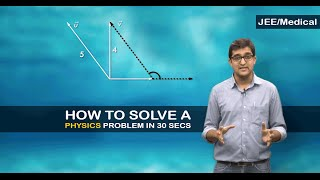# Pulley Problems

Here we will look at some of the problems based on the pulley and learn how to solve them. Well also understand a few equations involved and how we can apply them in solving different problems.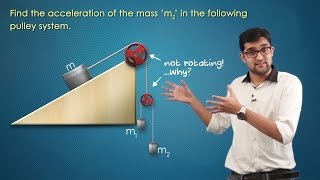## How To Solve Pully Problems?

For solving any pulley problem, the first step is to understand the given conditions and write down the constraint equations accordingly.

CASE – 1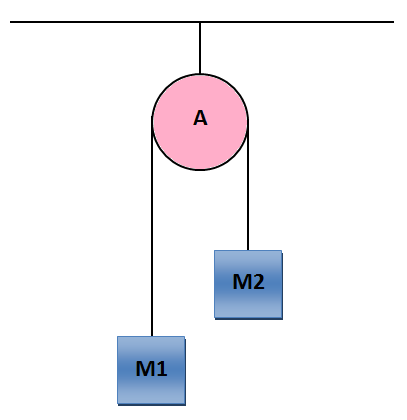Let, M1 & M2 be the mass attached to the pulley A. Now, consider that the mass M1 is moving down with acceleration a1 and mass M2 is moving up with acceleration a2.

Now, from the Free Body Diagram (FBD):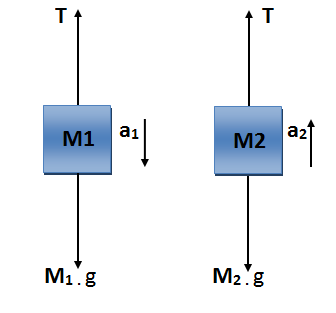The equation of force from FBD:

M1.g – T = M1.a1 . . . . . . . . . . (1)

T – M2.g = M2.a2 . . . . . . . . . . . (2)

The equation of force from FBD:

M1.g – T = M1.a1 . . . . . . . . . . (1)

T – M2.g = M2.a2 . . . . . . . . . . . (2)

From the above force equation, we have three unknowns but there are only 2 equations (Equation (1) & Equation (2) ) so we need a third equation relating the two unknowns. The relation is known as constraint equation because the motion of M1 and M2 is interconnected.

The following assumptions must be considered before writing the equation:

1. The string is taut and inextensible at each and every point of time.

2. The string is massless and hence the tension is uniform throughout.

3. Pulley is massless.

The string is inextensible hence the total change in length of the string should be zero. Suppose mass M1 goes down by x1 distance and mass M2 moves up by x2 distance. Then, change in length = x1 – x2 (Taking elongation as positive and compression as negative)

On equating it to zero we get, x1 – x2 = 0 (x = displacement)

On Differentiating equation (3) twice we get,

a1 – a2 = 0 . . . . . . . . . (3) (a = acceleration)

Using Equation (1), (2) & (3) we can calculate the values of T, a1& a2. This is an example of a Fixed Pulley System.

CASE – 2

Consider the following pulley system: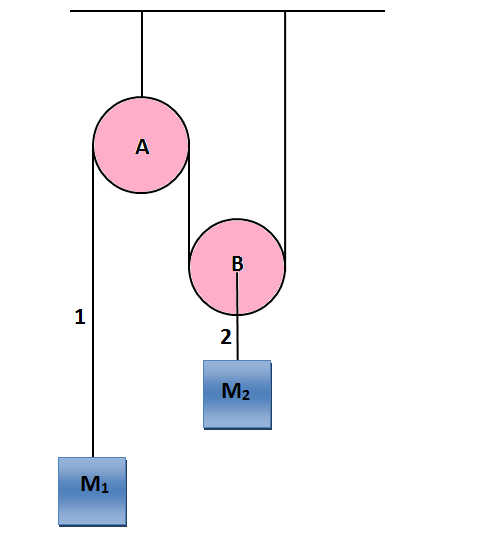First, we have to relate the tension between string 1 & string 2, Consider F.B.D of pulley B:2T1 – T 2 = MB.aB

2T1 = T2 . . . . . . . . . (4) (Since the pulley is massless)

Now, Consider the accelerations as shown in the below figure:For String 2:

Movable pulley B and Block M2 will have same acceleration otherwise the string will stretch or compress.

aB = a2 . . . . . . . . . . . . (5)

Increase in length to the left of fixed pulley A = L1

Decrease in length to the right of fixed pulley A = L2

Decrease in length to the right of movable pulley B = L3

And, L1 + L2 + L3 = Constant.

Therefore, the total change in length = L1 – 2L2 (Since, L2 = L3)

On differentiating the above equation twice we get,

$\Rightarrow$ a1 = 2a2 . . . . . . . . (6)

Similarly if we write force equation on both the blocks:

M1.g – T = M1.a1 . . . . . . . . . . (7)

2T – M2.g = M2.a2 . . . . . . . . . (8)

Using Equations (6), (7) & (8) we get:

T = $\frac {3 M_1 M_2 g}{4M_1~ +~ M_2}$

a1 = $\frac {(4M_1 ~-~ 2 M_2)g}{4M_1 ~+~M_2}$

a2 = $\frac {(2 M_1~-~M_2)g}{4 M_1~+~M_2}$

Instead of using the above method we can also use a shortcut to find the constraint relationship for saving time in JEE.

If we consider all the block + Pulleys + string as one system then the tension becomes internal force and we know that the work done by the internal force is zero.

$\Rightarrow$ $\sum T.x$ = 0

Work done by tension on block M1 = -T.x1

Work done by tension on block M2 = 2T.x2

Work by tension on pulley A = T.0 = 0

Work done by tension on Pulley B = 2T.x2 – 2T.x2 = 0

Hence we get, 2T.x2 – T.x1 = 0

$\Rightarrow$ x1 = 2.x2

Differentiating twice with respect to time we get:

$\Rightarrow$ a1 = 2.a2

The above technique can be used in almost all possible pulley system consisting of fixed pulleys or movable pulleys.